The calculators on this page require JavaScript but you appear to have switched JavaScript off (it is disabled). Please go to the Preferences for this browser and enable it if you want to use the calculators, then Reload this page.
start

If you want to explore runsums for yourself, try the Introduction to Runsums page at this site first.
This page summarizes some of the known results on runsums, provides tables, formulae and links to other sources of reference.
The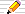icon means there is a You do the maths section of questions to start your own investigations. The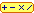calculator icon indicates that there is a live interactive calculator in that section.

## Definitions

Run
a series of consecutive (whole) numbers of any length such as 4,5,6,7.
A single number is also a run.
Sum(a,b)
The sum of the whole numbers from a to b, including those two numbers, is
sum(a,b) =
 (b – a + 1)(a + b)2
= number of values × average value

where the average value is (a + b)/2 and
the number of values is b – a + 1
R(n)
The number of runsums of n
E.g.: 15 = 7+8 = 4+5+6 = 1+2+3+4+5 so R(15) = 4
Runsum
Trapezoid
another name for a runsum. This name arises because the series sum can be shown as a trapezium made of a triangular number with a triangle removed from the top:
4+5+6 = 15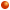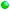15
= 1+2+3+4+5+6(1+2+3)
= T(6) – T(3)
This illustrates that
all runsums (trapezoids) are the difference of two triangular numbers.
Note that the second triangular number can be T(0)=0 so trapezoids includes triangles too!
Triangular number
a runsum which starts at 1; the sum of the series 1+2+3+...+n. The name arises because we can make a triangle of dots out of a "triangular number" of dots, starting with 1 at the top and each row one longer than the row above it:
e.g.
n123456789101112
T(n) as a sum11+21+2+31+2+3+4...
T(n) as a triangle...
T(n)=136101521283645556678
1,3,6,10,15,... is A000127
T(n)
T(n) = 1+2+3+ ...+n = n(n+1)/2 is the nth Triangular number
Here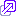are a couple of proofs of this formula.

### Runsum and Triangular Number Calculator

C A L C U L A T O R
Runsums: Find a run's SUM
the sum of all the whole numbers from up to
Runsums: Find a run from a SUM
 all shortest longest runs with a sum of up to
 with sum starting ending at runs
Triangular Numbers:
T() up to T()
R E S U L T S

## Formulae

T(n) = n(n+1)/2

### The runsum from a to b

The number of dots in a trapezoid of a dots on the top row (a+1 on the next and so on) and b dots on the final row (with ba)
 a balls.. ..a+1 balls... ..................... b balls..... .....S(a,b) = a + (a+1) + (a+2) + ... + (b–1) + b = (1+2+...+(a–1)+a+...+b) – (1+2+...+(a–1)) = a(b) – a(a–1) = b(b + 1)/2 – (a – 1)a/2 = (b2 + b – a2 + a)/2 = (b – a + 1)(a + b)/2

Special case a=b: S(b,b) = 2b/2 = b
Special case a=0: S(0,b) = (b+1)b/2
Special case a=1: S(1,b) = b(1+b)/2 = S(0,b) which is also T(b), a triangular number

### The number of runsums of n

The formula for the number of runsums of n, R(n), depends on factorizing n into its prime factors. If prime number pi occurs as a factor of n a total of ei times then piei is a factor of n.
We distinguish between the prime factor 2 and the rest of the prime factors, which will, of course, all be odd numbers.
If n = 2cp1e1 p2e2 p3e3 ...
where all pi are prime numbers bigger than 2,
then R(n) = (e1+1)(e2+1)(e3+1)... is the number of runsums of n.

E.g.: 60 = 22×3×5 = 22×31×51 so
p1=3 and e1=1 and
p2=5 and e2=1.
So there are R(60) = (1+1)(1+1) = 4
The 4 runsums are 4+5+6+7+8+9+10+11 = 10+11+12+13+14 = 19+20+21 = 60.

#### Counting the number of runsums: Calculator

Count Runsums C A L C U L A T O R
 all shortest longest runs with a sum of up to
R E S U L T S

### Runsums of a given length

#### Runs of length 1, 2 and 3

For every number there is a runsum of just one number, a run of length 1, namely, the number itself!

Numbers that are the sum of a run of length 2 are of the form n+(n+1) = 2n+1, that is, every odd number is a runsum of length 2.
E.g. 5=2+3, 7=3+4, 9=4+5.

Runsums of length 3 are of the form n+(n+1)+(n+2) = 3n+3 = 3(n+1), so every multiple of 3 has a runsum of length 3 and we note that 3 = 0 + 1 + 2.
E.g. 6=1+2+3, 9=2+3+4, 27=8+9+10, and in general 3k=(k-1) + k + (k+1).

#### Runs of length>3

You can now see how to find a formula for a number which has a runsum of length 4, 5, 6, and so on.
To summarize:
N=1: Every number of the form  n   has a runsum of length 1 (i.e.every number!);
which are the numbers 1, 2, 3, 4, 5, 6, ...
N=2: Every number of the form 2n+ 1 has a runsum of length 2;
which are the numbers 3=1+2, 5=2+3, 7=3+4, 9=4+5, 11=5+6, 13=6+7, 15, ...
N=3: Every number of the form 3n+ 3 has a runsum of length 3;
which are the numbers 6=1+2+3, 9=2+3+4, 12=3+4+5, 15=4+5+6, 18=5+6+7, 21, 24, ...
N=4: Every number of the form 4n+6 has a runsum of length 4;
which are the numbers 10=1+2+3+4, 14=2+3+4+5, 18=3+4+5+6, 22=4+5+6+7, 26, 30, 34, ...
N=5: Every number of the form 5n+10 has a runsum of length 5;
which are the numbers 15=1+2+3+4+5, 20=2+3+4+5+6, 25=3+4+5+6+7, 30, 35, 40, ...
and so on.

#### General case

The general formula is that
for all numbers k, N k + T(N) has a runsum of length N
where T(N) is the N-th triangular number, i.e.
The numbers N k + N(N+1)/2 = N (2k + N + 1)/2 for any k≥1 all have a runsum of length N
The smallest number with a runsum of length N is 1+2+3+...+N=T(N). This follows from the definition of a triangular number T(L) as the sum of the first L numbers starting at 1.

#### Find Numbers with a runsum of a given length: Calculator

Runsums of a given length C A L C U L A T O R
 runs of length
R E S U L T S

## Tables

By making a table of values of runsums, we can spot patterns.
This Tabulator will display tables of runsums as numbers or highlighted colours between a range of runsum starting and ending values.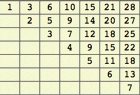Here is a table of runsums from Start to End, that is Start + (Start+1) + ... + (End-1) + End arranged in a table with Start ≤ End. The Starting values are down the left-hand edge and the Ending values go across the Table.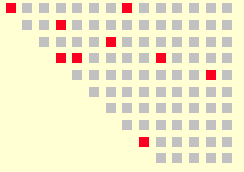For instance, here are the runsums that are square numbers (4-gonal numbers) shown as points with the square numbers highlighted.Another option is to look at the remainders when the runsums are divided by a given number (the modulo or mod). The remainders are each coloured or in greyscale, one per remainder, to help identify patterns.
Here is an example with 7 different greys for the remainders when runsums are divided by 7.

See more on triangular and other polygonal numbers.

### Runsums Tabulator

T A B U L A T O R
Make Tables of Runsum values
 ←ending→ ↑starting↓
 as numbers as points with highlights highlighting any that are: ≡ mod Oblong Numbers Primes -gonal numbers Centre -gonal numbers Fibonacci numbers General Fibonacci from , print the highlighted numbers also:
using
highlighting all remainders mod

R E S U L T S

All runsums of all numbers up to 10:
12345678910
121+2
3
42+3
5
1+2+3
6
3+4
7
82+3+4
4+5
9
1+2+3+4
10

### The number of runsums of n

Let R(n) be the number of runsums of n.
 n R(n) 1 2 3 4 5 6 7 8 9 10 11 12 13 14 15 16 17 18 19 20 1 1 2 1 2 2 2 1 3 2 2 2 2 2 4 1 2 3 2 2
This is series A001227 in Sloane's Encyclopaedia of Integer Sequences.
If we exclude single number runs, then we subtract one from each of the numbers in the series above and have Sloane's A069283.

### The least number with r runsums

Let Le(r) be the least number with exactly r runsums, i.e. Le(r) = i means R(i)=r and i is the smallest.
 n Le(n) andfactors n Le(n) andfactors 0 1 2 3 4 5 6 7 8 9 1 3 9=32 15=3x5 81=34 45=32x5 729=36 105=3x5x7 225=32x5210 11 12 13 14 15 16 17 18 19 405=34x5 59049=310 315=32x5x7 531441=312 3645=36x5 2025=34x52 945=33x5x7 43046721=316 1575=32x52x7 387420489=318
This is series A038547 in Sloane's Encyclopaedia of Integer Sequences.
It contains some interesting patterns that depend on factoring the number n into a product of numbers and using those factors each decreased by 1 as powers of the odd prime numbers in order: 3,5,7,11,13,17,....

#### Least number with R runsums Calculator

C A L C U L A T O R
 with runsums
R E S U L T S

### Numbers with an odd number of runsums

You might have noticed that most numbers in the table of The Number of runsums of n are even. The exceptions are the powers of 2 which we know are unique in that they have only 1 runsum that consists of just the number n itself.
The least number with r runsums, above, shows that we can find at least one number with r runsums for any r we choose. The method of doing this is given below in the Algorithms section.
So here is the start of the list of those comparatively rare numbers with an odd number of runsums (there are only 53 such numbers less than 1000):
 n Number ofrunsums of n 1 2 4 8 9 16 18 25 32 36 49 50 64 72 81 98 100 ... 1 1 1 1 3 1 3 3 1 3 3 3 1 3 5 3 3 ...
This is series A028982 in Sloane's Encyclopaedia of Integer Sequences. It is described there as the combination of two separate sequence of numbers : the square numbers (1, 4, 9, 16, 25, ...) and numbers that are twice a square number (2, 8, 18, 32, 50, ...).

### A special series of runsums - with Triangle number limits

Suppose we write all the whole numbers in rows with 1 on the first row, the next 2 numbers on the second, the next 3 on the third and so on, as follows, finding the sum of each run in the rows:
Rowrunrunsum
1:11
2:2 35
3:4 5 615
4:7 8 9 1034
5:11 12 13 14 1565
.........
This series of runsums in red can be described by a simple formula. If we first double each runsum and then take away the row number we get a series you may recognise:
RRow Rrunsum2 runsum - R
1:112x1 – 1 = 1
2:2 352x5 – 2 = 8
3:4 5 6152x15 – 3 = 27
4:7 8 9 10342x34 – 4 = 64
5:11 12 13 14 15652x65 – 5 = 125
............
The green numbers in the final column are the cubes of the row number, i.e. R×R×R = R3:
13=1, 23=8, 33=27, 43=64, 53=125, ...
So the runsum in the row R of the table is the cube R3 plus half the row number R or:
Row R is a runsum of (R3 + R)/2

#### Can we describe the runs themselves?

What is the run on row 10? or row 100? Can we find it directly without finding all the runs on all previous rows?
There is a simple formula. Try to spot a pattern yourself, if you like, before reading on.

Do you notice anything special about the final number on each row?

Rowrunrunsum
1:11
2:2 35
3:4 5 615
4:7 8 9 1034
5:11 12 13 14 1565
.........
You should do by now ... they are our old friends the Triangle numbers:
1, 3, 6, 10, 15, ... where T(R)= R(R+1)/2
You should therefore be able to tell what number begins row R.
If the previous row, R-1, ends with T(R-1), then row R begins with the next number: T(R-1)+1.
So row R is the run from T(R-1)+1 up to T(R).

In our table, row R:

 The sum of the run of R numbers from T(R-1)+1 ending with T(R) is (R3 + R)/2
This is Sloane's series A006003.

### Another special series of runsums - with Square number limits

The first special case, above, has a run of length 1 on row 2, of length 2 on row 2, and so on.
This time, we'll have a runs of lengths 1,3,5,7 and so on on each row, again just listing the integers one after the other, continuing on the next row when we finish one row...let's see what happens:
Rowrunrunsum
1:11
2:2 3 49
3:5 6 7 8 935
4:10 11 12 13 14 15 1691
5:17 .. 25189
.........
This time we notice that each row ends with a square number 1, 22=4, 32=9, 42=16, ...
What about the run on row R?
• row R ends with R2
• row R must start with the number after the one that row R-1 ends with;
• row R-1 ends with (R-1)2;
• therefore row R starts with (R-1)2+1
So Row R is the run (R-1)2+1 .. R2

As a hint, notice that the sum on row 2 is 9 = 1+8 and the sum on row 3 is 35 = 8+27.
You might remember that 1, 8 and 27 are the cubes 13=1, 23=8 and 33=27.

• How would you continue this pattern to row 4?
• What sum might you expect?
• Are you correct?
This time we guess that row R is:
 The sum of the run of 2R–1 numbers from (R-1)2+1 to R2 is R3 + (R-1)3
The exercise below leads you to a proof.

This is Sloane's A005898 also called the Centred Cubic numbers since they are the numbers of dots in a cube of side 1, then surround it by a cube of 2 dots on each side, then another outer coating of a cube of 3 dots per side (and 9 per face), and so on. There is a nice animation of this, which you can manipulate with your mouse on Eric Weisstein's World Of Mathematics page on the Centered Cube Numbers (with the US spelling this time).

#### You do the maths...

1. Using the formula for T(R) = R(R+1)/2, prove that T(R2) – T((R–1)2) describes the runsum on row R and show that the result is the same as R3 + (R-1)3
8 T(T(R)) = 2R + 3R2 + 2R3 + R4
8 T(T(R−1)) = −2R + 3R2 − 2R3 + R4
8 T(T(R)) - 8 T(T(R−1)) = 4R3 + 4R
2 T(T(R)) - 2 T(T(R−1)) = R3 + R

## Algorithms

An algorithm is a description of a method of doing something that is detailed enough for a computer to execute. They are therefore precise and full enough to be made into a program in a particular computer programming language such as Java or C or even using a spreadsheet such as Excel. A formula, such as T(n) = n(n+1)/2 for the n-th triangular number, is a simple kind of algorithm, since we just plug in a number into the formula and the answer is found by arithmetic calculation.

### Finding runsums of a given length

We have a description above for all the numbers with a runsum of length L:
Lk+T(L) for k=0,1,2,3,...
i.e. T(L), L+T(L), 2L+T(L), 3L+T(L), ...
By this method we can test any number n to see if it has a runsum of length L for all the various L's as so we will find all the runsums for n.

#### What is the maximum length of a runsum of n?

The smallest number with a runsum of length L is T(L). So the longest runsum (of length m) of n will be the largest m for which T(m) is less than (or equal to) n.
E.g. Using the Table of Triangular numbers above, for n=50, the largest triangular number less than or equal to 50 is T(9)=45.
So we test all L from 1 to 9 and see if there is a runsum of length L for our number n.

#### Is there a runsum of length L for n?

If there is a runsum of length L for our number n, then n will be in the list
T(L), L+T(L), 2L+T(L), 3L+T(L), ..., kL+T(L), ... for some k = 0,1,2,3...
Since we know T(L), we can subtract T(L) from n. If what is left is of the form k L (for some k) then there is a runsum of length L for n. It is easy to test if what is left is a multiple of L. We divide L into it and look at the remainder. Most calculators and most programming languages have built-in function to find the remainder after a division. It is called mod, the modulo function.
The remainder on dividing n by L is written n mod L.
E.g.
15 mod 7 = 1 since there is 1 left over when we divide 7 into 15.
15 mod 6 = 3 since 6 goes into 15 twice with a remainder of 3.
15 mod 5 = 0 since 5 goes exactly into 15 so there is no remainder.
If a mod b = 0 then a is divisible exactly by b.
Here are some examples to find some specific runsums for n=11:
Is there a runsum of length L=3 ?
T(L)=T(3)=6 which we subtract from 11 to get 5. Is 5 a multiple of L=3? No since 5 mod 3 = 2. So there is no runsum of length 3 for 11.
Is there a runsum of length 2?
T(L)=T(2)=3 which we subtract from 11 to get 8. Is 8 a multiple of L=2? Yes because 8 mod 2 = 0. So there is a runsum of length 2 for 11 (and a bit of thought shows that it is 5+6).

### Finding a runsum of length L for n

So once we know there is a runsum for n of length L, how do we find it?
The formula for a runsum of length L tells us how.
A runsum of length L is of the form
(k+1) + (k+2) + ... + (k+L).
This sum has L numbers in it, each in a bracket, each bracket having a red and a blue part. We find the sum of the L bracketed parts by summing the red parts and the blue parts separately.
There are L lots of k, giving Lk
The blue parts are 1+2+3+...+L which is just T(L).
So if there is a runsum of length L for n, then
k tells us what to add to each of the numbers in 1+2+...+L to get the runsum of length L for n.

k = (n-T(L))/L

E.g.. Find a runsum of length L= 2 for n=11.
From the example in the previous section, we found that there is a runsum of length L=2 for n=11.
11 - T(2) = 11-3 = 8. 8 is a multiple of L=2 FOUR times so k=4.
So the runsum is 1+2 (the smallest runsum of length L=2) but with k=4 added to each part of it:
(1+4) + (2+4) i.e.5+6. Checking 5+6 is indeed 11, our n!

E.g.. Find a runsum of length L= 5 for n=35.
T(5)=15. n-T(5) is 35-15 = 20 which is a multiple of L=5. The multiple is 4 (4x5=20) so k=4.
The runsum is therefore the smallest runsum of length 5, 1+2+3+4+5, with k=4 added to each part of it:
35 = 5+6+7+8+9.

### Finding all the runsums of a given number

Now we know the longest runsum that n can have (the largest L such that T(L) is less than or equal to n) and we know how to find the runsum of length L, if one exists, for n. This is now a complete method for finding all the runsums of n: it is an algorithm for generating the runsums of n.

```     Find the largest number L for which T(L) is less than or equal to n.
Call this value M.
For each value of L from 1 to M:
see if there IS a runsum of length L for n
and, if there is,  find the runsum as we did above and print it out
```

E.g.: Find all the runsums of 33. T(7)=28 and T(8)=36, there are no runsums of length 8 or more for n=33.
So we try L = 1, 2, 3, 4, 5, 6, 7 in turn:
L=1: This is a special case as we know every number has the trivial runsum of length 1.
Our method above still works though:
• L=1:
T(1)=1 and 33-1=32 which is a multiple of L=1. The multiple (k) is 32. So we add 32 to each part of the smallest runsum of length 1, namely 1, to get 1+32 or 33 on its own.
• L=2:
T(2)=3 and 33-3=30 which is a multiple of L=2. The multiple is 15, so we add 15 to each part of 1+2 and get 16+17=33.
• L=3:
T(3)=6 and 33-6=27 which is 9=k times 3=L. So the runsum of length 3 for 33 derived from 1+2+3 is 10+11+12.
• L=4:
T(4)=10 and 33-10=23 is not a multiple of L=4.
• L=5:
T(5)=15 and 33-15=18 which is not a multiple of L=5.
• L=6:
T(6)=21 and 33-21=12 which is 2=k times 6=L. So the runsum of length 6 for 33 derived from 1+2+3+4+5+6 is 3+4+5+6+7+8.
• L=7:
T(7)=28 and 33-28=5 which is again not a multiple of 7.
So we have found 4 runsums of 33: 33; 16+17; 10+11+12; 3+4+5+6+7+8.

## More Puzzles and Problems

### You do the maths...

1. Examine the runsums from n up to 2n–1 for n from 1 upwards.
2. How many numbers are there in each of these runsums?
3. Find a formula for the sum of the numbers in the run from n to 2n–1.
4. What is another name for this series of runsums?
1 = 1
2 + 3 = 5
3 + 4 + 5 = 12
4 + 5 + 6 + 7 = 22
5 + 6 + 7 + 8 + 9 = 35
...
1, 5, 12, 22, ... are the Pentagonal numbers = n(3n-1)/2 A000326
1. Look at the runsums of 1, 6, 15, 28, 45, 66, 91, 120,... .
1. Describe this series in a mathematical formula.
2. Describe the runsum pattern common to each of the numbers in this series.
3. Prove by algebra that your answers to parts a and b are equal.
Runsums from 1 to 2n-1:
n=1: 1 = 1
n=2: 1 + 2 + 3 = 6
n=3: 1 + 2 + 3 + 4 + 5 = 15
n=4: 1 + 2 + 3 + 4 + 5 + 6 + 7 = 28
...
1, 6, 15, 28, 45, 66, 91, ... n(2n-1), ... A000384 are the Hexagonal Numbers
2. Examine the runsums from 2n+1 to 3n+1.
1. Write out the first 8 runsums in the form I..J replacing I and J by numbers.
3. Find an algebraic formula for the runsum values and prove it is correct.
3 + 4 = 7
5 + 6 + 7 = 18
7 + 8 + 9 + 10 = 34
9 + 10 + 11 + 12 + 13 = 55
... 1,7,18,34,55,81, .. n(5n-3)/2, ... A000566 are the Heptagonal Numbers
3. Find a pattern of runsums for the sums 1×3, 2×5, 3×7, 4×9, 5×11, ...
1 + 2 = 3 = 1×3
1 + 2 + 3 + 4 = 10 = 2×5
1 + 2 + 3 + 4 + 5 + 6 = 21 = 3×7
1 + 2 + ... + 7 + 8 = 36 = 4×9
1 + 2 + ... + 9 + 10 = 55 = 5×11
...
1 + 2 + ... + 2n = n(2n+1)
3, 10, 21, 36, 55, 78, ... A014105
are called the Second Hexagonal Numbers -- compare this with the answer to Question 2 above

## References

• On Representation as a Sum of Consecutive Integers W J Leveque Canad J. Math. vol 4 (1950) pages 399-405.
• Sums of Consecutive Integers R Guy Fibonacci Quarterly 20 (1982) pages 36-38 provides formulae for the number of ways to write n as a runsum of even and of odd length.

 © 1996-2018 Dr Ron Knott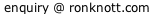updated 30 January 2018

Back to the Introduction to Runsums page
Back to the main (Fibonacci) page